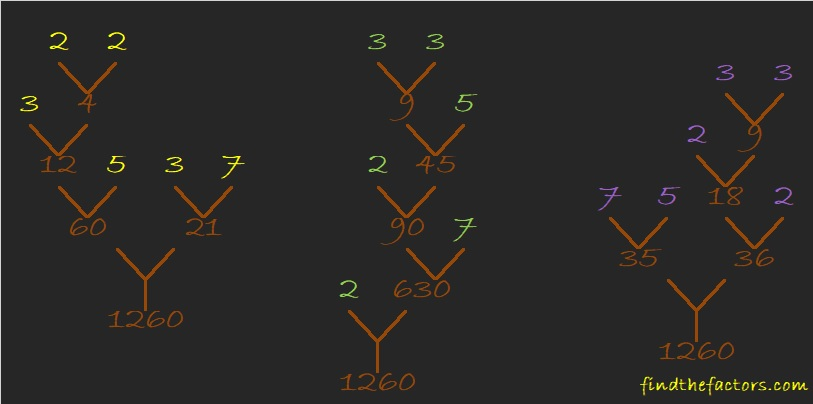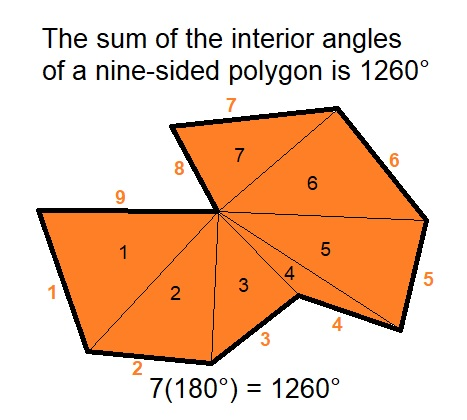# Haunted Forest with 1260 Factor Trees

1260 is the smallest number with 36 factors. That’s a new record. (32 was the old record and was held by both 840 and 1080.)

Often when a number has a lot of factors, we will visit a forest of its factor trees. 1260 certainly deserves such a forest. Since it is just before Halloween, It happens to be a haunted forest. Do you dare to go into such a forest? These three trees are scary enough for me! However, there are MANY more factor trees in that haunted forest! Perhaps if you are brave, you can find some of those factor trees in the haunted forest yourself.Here’s more about the number 1260:

• 1260 is a composite number.
• Prime factorization: 1260 = 2 × 2 × 3 × 3 × 5 × 7, which can be written 1260 = 2² × 3² × 5 × 7.
• The exponents in the prime factorization are 2, 2, 1, and 1. Adding one to each and multiplying we get (2 + 1)(2 + 1)(1 + 1) (1 + 1) = 3 × 3 × 2 × 2  = 36. Therefore 1260 has exactly 36 factors.
• Factors of 1260: 1, 2, 3, 4, 5, 6, 7, 9, 10, 12, 14, 15, 18, 20, 21, 28, 30, 35, 36, 42, 45, 60, 63, 70, 84, 90, 105, 126, 140, 180, 210, 252, 315, 420, 630, 1260
• Factor pairs: 1260 = 1 × 1260, 2 × 630, 3 × 420, 4 × 315, 5 × 252, 6 × 210, 7 × 180, 9 × 140, 10 × 126, 12 × 105, 14 × 90, 15 × 84, 18 × 70, 20 × 63, 21 × 60, 28 × 45, 30 × 42 or 35 × 36
• Taking the factor pair with the largest square number factor, we get √1260 = (√36)(√35) = 6√35 ≈ 35.4964821 × 60 = 1260 The same digits are used on both sides of that equation and that makes 1260 the 19th Friedman number.

1260 is also the sum of the interior angles of a nine-sided polygon. Convex or concave, that is the sum. The concave nonagon below is a good illustration of that fact:1260 is also the hypotenuse of a Pythagorean triple:
756-1008-1260 which is (3-4-5) times 252

This site uses Akismet to reduce spam. Learn how your comment data is processed.